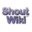Sayahna

## Template:Probability distributions/doc

Use one of the following depending on the transcluding article. This will show all related articles while hiding the less relevant ones.

`{{Probability distributions|discrete-finite}}`
`{{Probability distributions|discrete-infinite}}`
`{{Probability distributions|continuous-bounded}}`
`{{Probability distributions|continuous-semi-infinite}}`
`{{Probability distributions|continuous-infinite}}`
`{{Probability distributions|continuous-variable}}`
`{{Probability distributions|mixed}}`
`{{Probability distributions|multivariate}}`
`{{Probability distributions|directional}}`
`{{Probability distributions|miscellaneous}}`
`{{Probability distributions|families}}`